Pandas Dataframe: Merge and Join Examples

# Pandas Dataframe: Merge and Join Examples

Last updated:

WIP Alert This is a work in progress. Current information is correct but more content may be added in the future.

View examples on this jupyter notebook

## pd.merge example

The default is an inner join. Use 'how'='left'|'right'|'outer' to change join types.

import pandas as pd

pd.merge(
left_dataframe,
right_dataframe,
left_on= column_on_left_dataframe,
right_on= column_on_right_dataframe)


## pd.merge by index

Joins by index are much faster than join on arbitrary columns!

If the columns you want to join on are Indices, use left_index and right_index.

Use 'how'='left'|'right'|'outer' to change join types. The default join type is "left":

pd.merge(
left_dataframe,
right_dataframe,
left_index=True,
right_index=True)


## Join on multiple columns

Joining by multiple columns is useful for dealing with time-stamped data.

Just pass an array of column names to left_on and right_on:

import pandas as pd

df_employees_sal = pd.DataFrame({
'year':[1980,1981,1980,1981,1980,1981,1980,1981],
'id':[1,1,2,2,3,3,4,4],
'name':['alice','alice','bob','bob','charlie','charlie','david','david'],
'salary':[30000,30000,40000,41000,35000,40000,45000,45000],
'company_id':[1,1,2,2,1,1,2,2]})

df_companies_rev = pd.DataFrame({
'year':[1980,1981,1980,1981],
'id':[1,1,2,2],
'name':['bell labs','bell labs','xerox','xerox'],
'revenue':[1130000,1130000,5000000,500000]})

pd.merge(
df_employees_sal,
df_companies_rev,
left_on=['year','company_id'],
right_on=['year','id']
)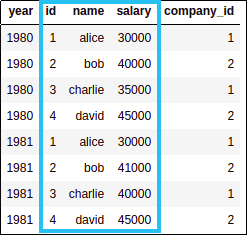LEFT: employees and
annual salaries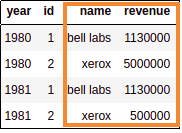RIGHT: companies and
annual revenue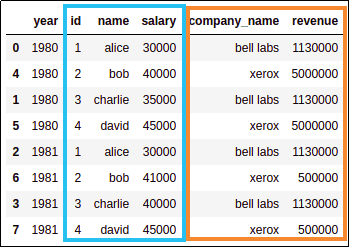MERGED using year and company_id as keys

## merge vs join

Joining by index (using df.join) is much faster than joins on arbtitrary columns!

The difference between dataframe.merge() and dataframe.join() is that with dataframe.merge() you can join on any columns, whereas dataframe.join() only lets you join on index columns.

## pd.merge() vs dataframe.join() vs dataframe.merge()

TL;DR: pd.merge() is the most generic. df.merge() is the same as pd.merge() with an implicit left dataframe. Use df.join() for merging on index columns exclusively. df.join is much faster because it joins by index

These are three different ways to do merging/joining dataframes on pandas:
pandas.merge dataframe.join dataframe.merge
How to callPandas global methodDataframe methodDataframe method
Join onJoin on any columnJoin on index columns onlyJoin on any column
PerformanceSlow unless using indicesFast!Slow unless using indices
Examplepd.merge(left_df, right_df)left_df.join(right_df)left_df.merge(right_df)
Equivalent to
(i.e. how to write it
using pd.merge)
-
pd.merge(
left_df,
right_df,
left_index=True,
right_index=True)
pd.merge(
df_left,
df_right)

## Rename duplicate columns

Pass suffix=(<suffix_for_left>,<suffix_for_right>) to pd.merge():

import pandas as pd

df_employees_sal = pd.DataFrame({
'year':[1980,1981,1980,1981,1980,1981,1980,1981],
'id':[1,1,2,2,3,3,4,4],
'name':['alice','alice','bob','bob','charlie','charlie','david','david'],
'salary':[30000,30000,40000,41000,35000,40000,45000,45000],
'company_id':[1,1,2,2,1,1,2,2]})

df_companies_rev = pd.DataFrame({
'year':[1980,1981,1980,1981],
'id':[1,1,2,2],
'name':['bell labs','bell labs','xerox','xerox'],
'revenue':[1130000,1130000,5000000,500000]})

# suffixes takes a tuple with the suffix values for duplicate columns coming
# from the left and right dataframes, respectively
pd.merge(
df_employees_sal,
df_companies_rev,
left_on=['year','company_id'],
right_on=['year','id'],
suffixes=('_left','_right')
)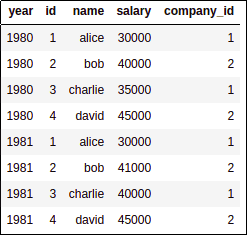LEFT: employess and
annual salariesRIGHT: companies and
annual revenue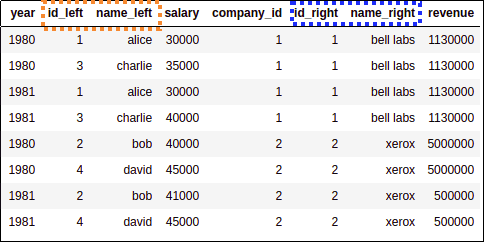MERGED: Newly named columns are left (orange) and right (blue)
Note that column year was not duplicated,
pandas correctly identified it
was the same in both sides.

TODO